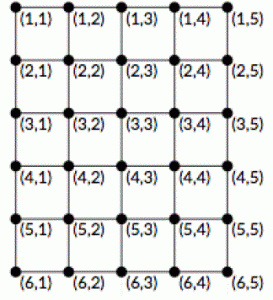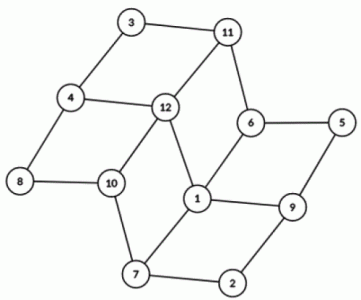favorite We need a little bit of your help to keep things running, click on this banner to learn more
Competitions

# Ancient Greek isomorphism

It is well known that the favourite graph of Zeus is a grid (namely, a rectangular grid graph) n * m, i.e. an undirected graph of n * m vertices and n * (m - 1) + (n - 1) * m edges, which can be represented as a grid with n rows and m columns so that each vertex is connected to neighbouring vertices.

Denote by (i, j) the vertex at the intersection of the i-th row and j-th column. Formally, in the graph there are edges between (i, j) and (i + 1, j) for all 1i < n, 1jm, and edges between (i, j) and (i, j + 1) for all 1in, 1j < m.(The illustration shows an example of a grid graph 6 * 5)

And surprisingly, it turned out that the beloved graph of Poseidon is also non-oriented, it also contains n * m vertices and n * (m - 1) + (n - 1) * m edges, and also does not contain loops and multiple edges. The vertices of his graph are numbered with integers from 1 to n * m.

Poseidon became interested, but maybe his and Zeus' graph are exactly the same (isomorphic)?

Formally, he wondered if its possible to find a unique correspondence between the vertices of the Poseidon graph and the vertices of the Zeus graph (that is, each vertex of the Poseidon graph should be associated with exactly one vertex of the Zeus graph so that each vertex of the Zeus graph was mapped to exactly one vertex of the Poseidon graph) so that two vertices are adjacent in one of these graphs if and only if their corresponding vertices are adjacent in another graph.

Help Poseidon with his difficult task, check his graph and the graph of Zeus for isomorphism!

#### Input

First line contains two integers n and m (2n, m, n * m2 * 105), indicating the size of the grid.

In the next n * (m - 1) + (n - 1) * m lines the description of the edges Poseidon's graph is given. The i-th line contains two integers ui and vi (1ui, vin * m, uivi), denoting an edge connecting the corresponding vertices.

It is guaranteed that the Poseidon's graph does not contain multiple edges.

#### Output

If the graphs of Poseidon and Zeus are isomorphic, print "Yes". Otherwise, print "No".

#### Sample

The graph from the second example is shown in the picture:Time limit 1 second
Memory limit 128 MiB
Input example #1
2 2
1 2
2 3
3 4
4 2

Output example #1
No

Input example #2
4 3
2 9
2 7
5 9
5 6
7 10
1 7
1 6
1 9
1 12
6 11
10 12
11 12
8 10
4 8
4 12
3 4
3 11

Output example #2
Yes

Source 2018 Cycle of Internet Olympiads for schoolchildren, second team season olympiad, Basic nomination, October 20, Problem F